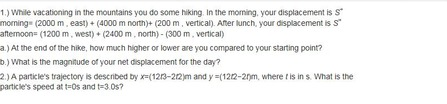# 1.) While vacationing in the mountains you do some hiking. In the morning, your displacement is S...

## Question:

1.) While vacationing in the mountains you do some hiking. In the morning, your displacement is {eq}\vec{S} {/eq} morning= (2000 m , east) + (4000 m north)+ (200 m , vertical). After lunch, your displacement is {eq}\vec{S} {/eq} afternoon= (1200 m , west) + (2400 m , north) - (300 m , vertical)

a.) At the end of the hike, how much higher or lower are you compared to your starting point?

b.) What is the magnitude of your net displacement for the day?

2.) A particle's trajectory is described by x=(12t3?2t2)m and y =(12t2?2t)m, where t is in s. What is the particle's speed at t=0s and t=3.0s?## Vector Algebra

An element of vector space in linear algebra is called a vector. This construct is characterized by a length (or magnitude) and an orientation in a given space (often the two- or three-dimensional space of our daily experience). Vectors can be added or multiplied with a scalar, the result of these operations is again a vector.

1a: This difference is given by the difference in altitudes which is {eq}\Delta h = \rm - \ 300~m \ - \ 200~m = - \ 500~m {/eq}. At the end of the...

Become a Study.com member to unlock this answer! Create your account